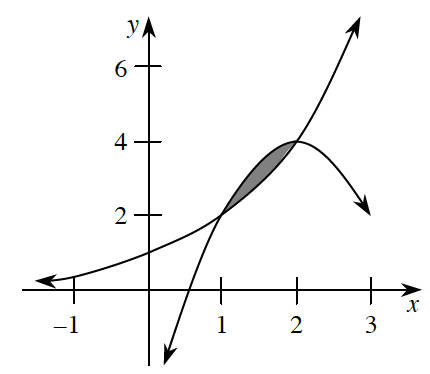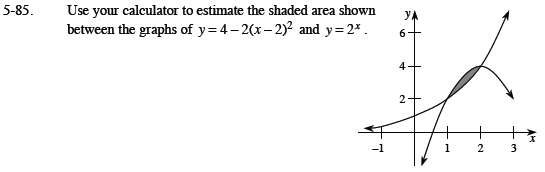### Home > CALC > Chapter 5 > Lesson 5.2.3 > Problem5-85

5-85.
1. Use your calculator to estimate the shaded area shown between the graphs of y = 4 − 2(x − 2)2 and y = 2x . Homework Help ✎Identify where the functions intersect, as well as which function is 'greater' (has higher y-values) than the other.

$\text{Integrate}: \int_{\text{intersection }A}^{\text{intersection }B}[\text{top function}]-[\text{bottom function}]dx$